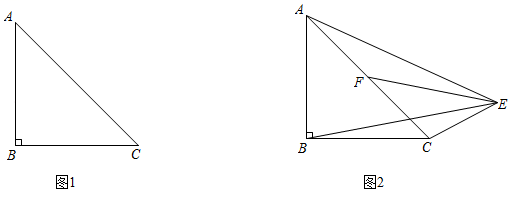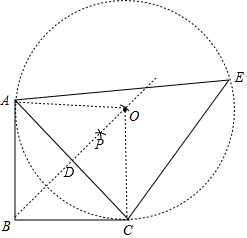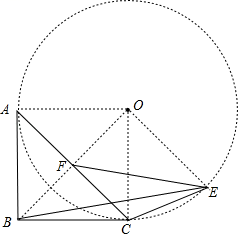(1) 利用尺规作图在图 1 中作出一个符合题意的点 $E$; (不写作法, 保留作图痕迹)
(2) 如图 2, 若 $F$ 是 $A C$ 的中点, 线段 $B E$ 与线段 $E F$ 的长度存在怎样的等量关系? 请说明理由.$\therefore$ 四边形 $A B C O$ 是菱形,
$\because \angle A B C=90^{\circ}$,
$\therefore$ 四边形 $A B C O$ 是正方形,
$\therefore \angle A O C=90^{\circ}=\angle A B C, O A=O C$,
$\therefore$ 点 $C$ 在圆 $O$ 上,
$\because \angle A O C=2 \angle A E C$,
$\therefore \angle A C B=2 \angle A E C$;

(2) $B E=\sqrt{2} E F$, 理申如下:$\because$ 四边形 $A B C O$ 是正方形, $F$ 是 $A C$ 的中点, $O A=O C$,
$\therefore O B$ 经过点 $F, \angle B C O=90^{\circ}, \angle C B O=\angle O C A=45^{\circ}, O F \perp A C$, 在 Rt $\triangle O B C$ 中,
$$\frac{O C}{O B}=\sin \angle O B C=\frac{\sqrt{2}}{2} \text {, }$$

\begin{aligned} & \frac{O \mathrm{~F}}{\mathrm{OC}}=\sin \angle O C A=\frac{\sqrt{2}}{2}, \\ & \therefore \frac{O C}{O B}=\frac{O F}{O C}=\frac{\sqrt{2}}{2}, \\ & \because O E=O C, \\ & \therefore \frac{O E}{O B}=\frac{O F}{O E}=\frac{\sqrt{2}}{2}, \\ & \because \angle E O F=\angle B O E, \\ & \therefore \triangle E O F \sim \triangle B O E, \\ & \therefore \frac{E F}{B E}=\frac{O F}{O E}=\frac{\sqrt{2}}{2}, \\ & \therefore B E=\sqrt{2} E F . \end{aligned}
①点击 首页查看更多试卷和试题 , 点击查看 本题所在试卷